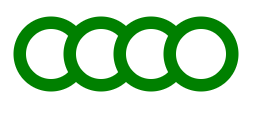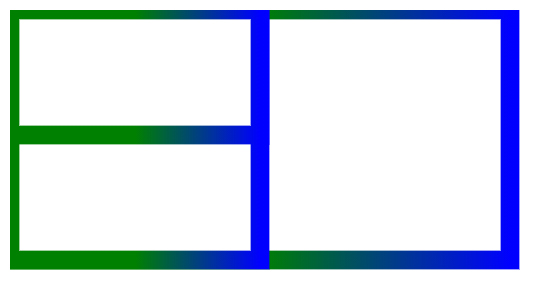# SVG <g> Element

• Last Updated : 16 Jun, 2022

The SVG <g> element is a container used to group other SVG elements.

Transformations applied to the <g> element are also performed on its child elements, and its attributes are inherited by its children.

Syntax:

```<g attributes="" >
<elements>
</g>```

Attributes:

• core Attributes: These attributes are core attributes like id, etc.
• styling Attributes: These attributes define styling, exp, class, style.
• conditional Attributes: These attributes are condition-based, exp systemLanguage.
• presentation Attributes: Attributes used to give presentation effects, exp color,clip-rule, etc.

Example 1: Making green consecutive circles inheriting attributes from the <g> element.

## html

 ```<``html``>` `<``body``>``    ``<``svg` `width="1200" height="1200">``        ``<``g` `fill="white" stroke="green" stroke-width="10">``            ``<``circle` `cx="40" cy="40" r="25" />``            ``<``circle` `cx="80" cy="40" r="25" />``            ``<``circle` `cx="120" cy="40" r="25" />``            ``<``circle` `cx="160" cy="40" r="25" />``        ````    ````` ``

Output:Example 2: Making rectangles with the same inherited properties.

## html

 ```<``html``>` `<``body``>``    ``<``svg` `width="1200" height="1200">``        ``<``defs``>``            ``<``linearGradient` `id="gfgStr">``                ``<``stop` `offset="50%"   stop-color="green" />``                ``<``stop` `offset="100%" stop-color="blue" />``            ````        ````      ` `        ``<``g` `fill="white" stroke="url(#gfgStr)" stroke-width="15">``            ``<``rect` `width="400" height="200" />``            ``<``rect` `width="200" height="200" />``            ``<``rect` `width="200" height="100" />``        ````    ````` ``

Output:Browsers Supported: The following browsers are supported by this SVG element:

• Chrome 1 and above
• Edge 12 and above
• Firefox 1.5 and above
• Safari 3.1 and above
• Internet Explorer 9 and above
• Opera 8 and above

My Personal Notes arrow_drop_up